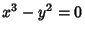## CuspA cusp is a point on a continuous curve where the tangent vector reverses sign as the curve is traversed. A functionhas a cusp (also called a Spinode) at a pointifis Continuous atandfrom one side whilefrom the other side, so the curve is Continuous but the Derivative is not. A cusp is a type of Double Point. The above plot shows the curve, which has a cusp at the Origin.

See also Double Cusp, Double Point, Ordinary Double Point, Ramphoid Cusp, Salient Point

References

Walker, R. J. Algebraic Curves. New York: Springer-Verlag, pp. 57-58, 1978.